Скачать презентацию Chapter 3 The Time Value of Money Part

e0dfeaab536068be10cfbaee401e5b1e.ppt

• Количество слайдов: 35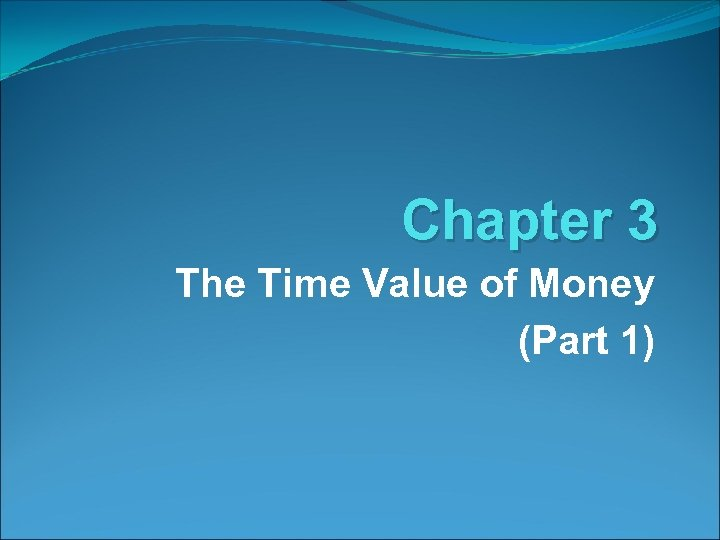Chapter 3 The Time Value of Money (Part 1)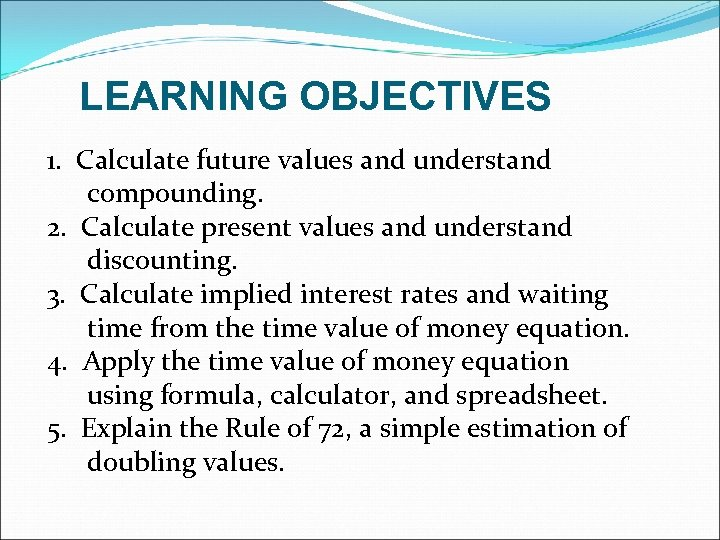LEARNING OBJECTIVES 1. Calculate future values and understand compounding. 2. Calculate present values and understand discounting. 3. Calculate implied interest rates and waiting time from the time value of money equation. 4. Apply the time value of money equation using formula, calculator, and spreadsheet. 5. Explain the Rule of 72, a simple estimation of doubling values.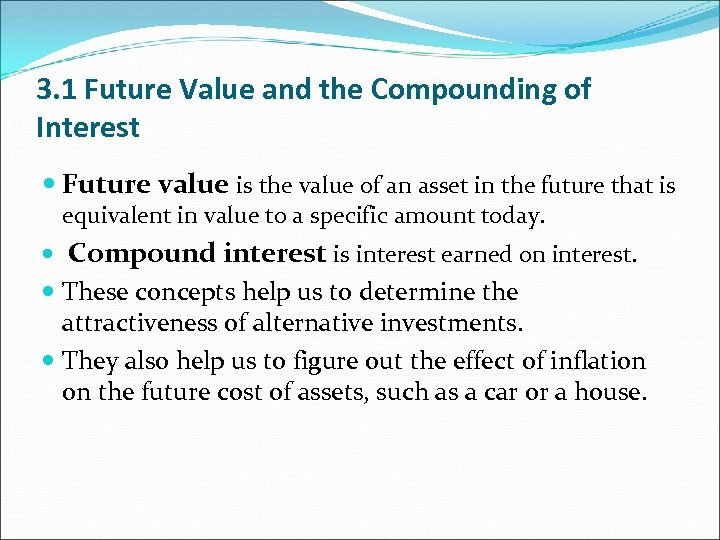3. 1 Future Value and the Compounding of Interest Future value is the value of an asset in the future that is equivalent in value to a specific amount today. Compound interest is interest earned on interest. These concepts help us to determine the attractiveness of alternative investments. They also help us to figure out the effect of inflation on the future cost of assets, such as a car or a house.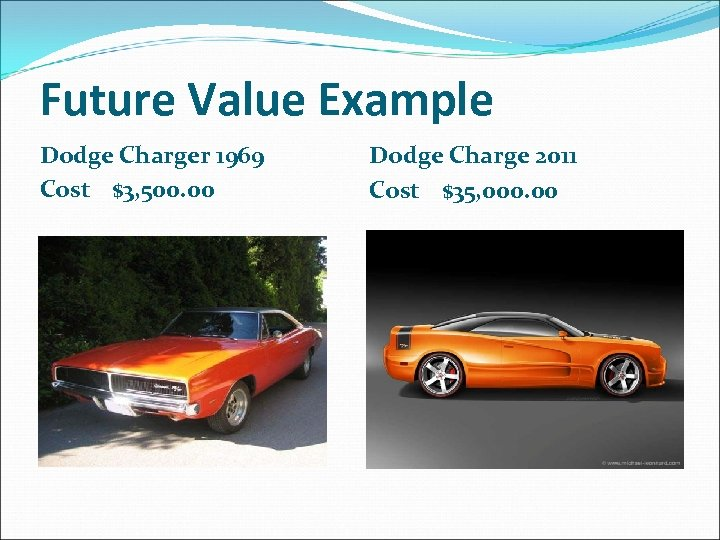Future Value Example Dodge Charger 1969 Cost \$3, 500. 00 Dodge Charge 2011 Cost \$35, 000. 00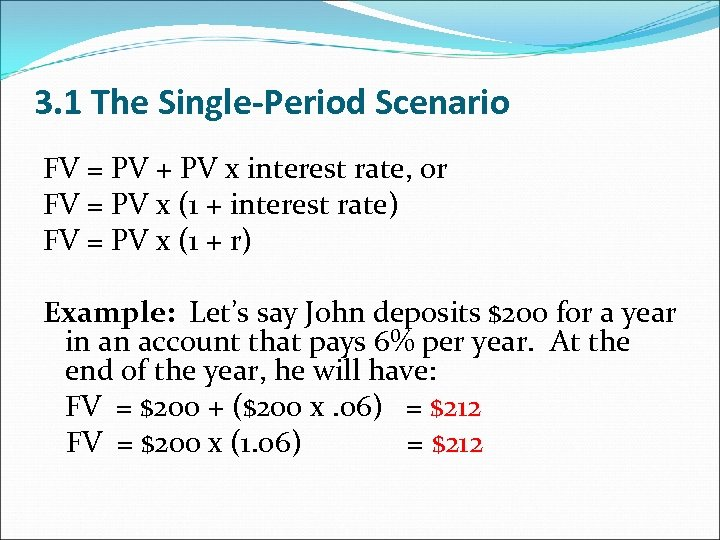3. 1 The Single-Period Scenario FV = PV + PV x interest rate, or FV = PV x (1 + interest rate) FV = PV x (1 + r) Example: Let’s say John deposits \$200 for a year in an account that pays 6% per year. At the end of the year, he will have: FV = \$200 + (\$200 x. 06) = \$212 FV = \$200 x (1. 06) = \$212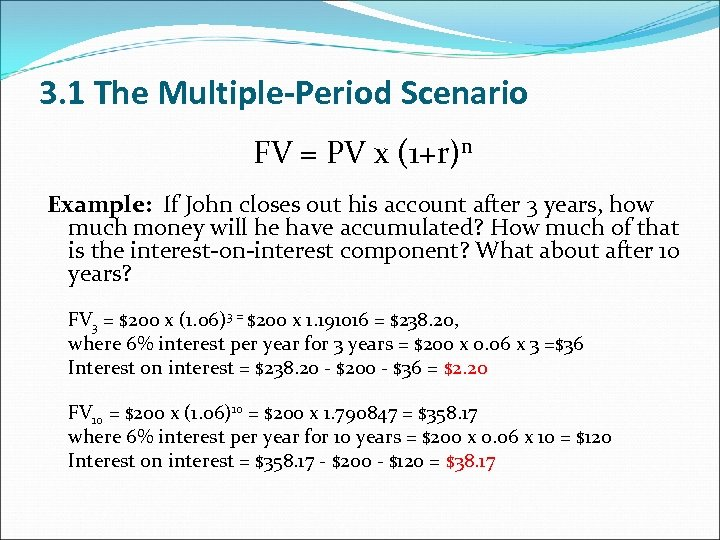3. 1 The Multiple-Period Scenario FV = PV x (1+r)n Example: If John closes out his account after 3 years, how much money will he have accumulated? How much of that is the interest-on-interest component? What about after 10 years? FV 3 = \$200 x (1. 06)3 = \$200 x 1. 191016 = \$238. 20, where 6% interest per year for 3 years = \$200 x 0. 06 x 3 =\$36 Interest on interest = \$238. 20 - \$200 - \$36 = \$2. 20 FV 10 = \$200 x (1. 06)10 = \$200 x 1. 790847 = \$358. 17 where 6% interest per year for 10 years = \$200 x 0. 06 x 10 = \$120 Interest on interest = \$358. 17 - \$200 - \$120 = \$38. 17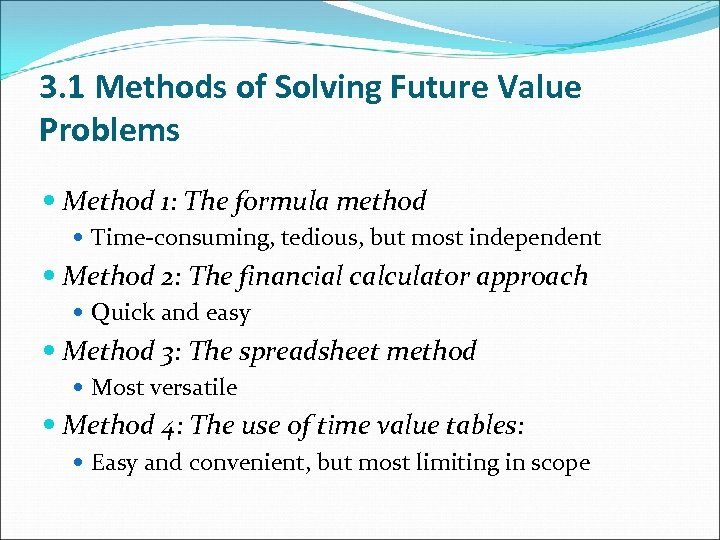3. 1 Methods of Solving Future Value Problems Method 1: The formula method Time-consuming, tedious, but most independent Method 2: The financial calculator approach Quick and easy Method 3: The spreadsheet method Most versatile Method 4: The use of time value tables: Easy and convenient, but most limiting in scope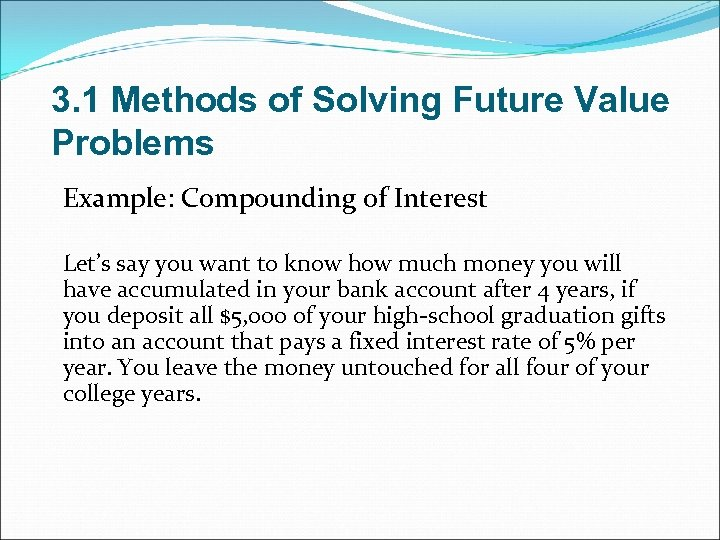3. 1 Methods of Solving Future Value Problems Example: Compounding of Interest Let’s say you want to know how much money you will have accumulated in your bank account after 4 years, if you deposit all \$5, 000 of your high-school graduation gifts into an account that pays a fixed interest rate of 5% per year. You leave the money untouched for all four of your college years.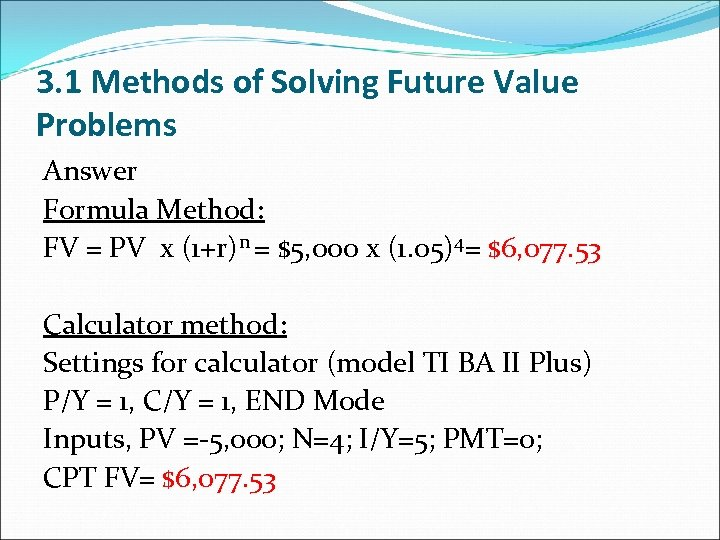3. 1 Methods of Solving Future Value Problems Answer Formula Method: FV = PV x (1+r)n = \$5, 000 x (1. 05)4= \$6, 077. 53 Calculator method: Settings for calculator (model TI BA II Plus) P/Y = 1, C/Y = 1, END Mode Inputs, PV =-5, 000; N=4; I/Y=5; PMT=0; CPT FV= \$6, 077. 53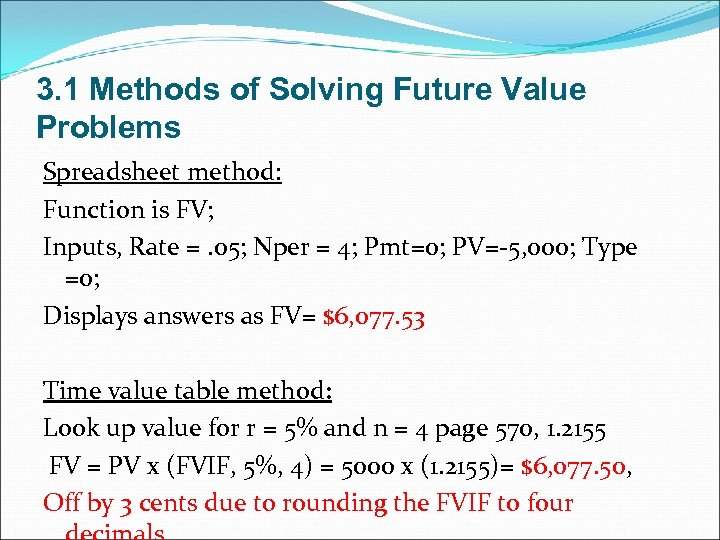3. 1 Methods of Solving Future Value Problems Spreadsheet method: Function is FV; Inputs, Rate =. 05; Nper = 4; Pmt=0; PV=-5, 000; Type =0; Displays answers as FV= \$6, 077. 53 Time value table method: Look up value for r = 5% and n = 4 page 570, 1. 2155 FV = PV x (FVIF, 5%, 4) = 5000 x (1. 2155)= \$6, 077. 50, \$6, 077. 50 Off by 3 cents due to rounding the FVIF to four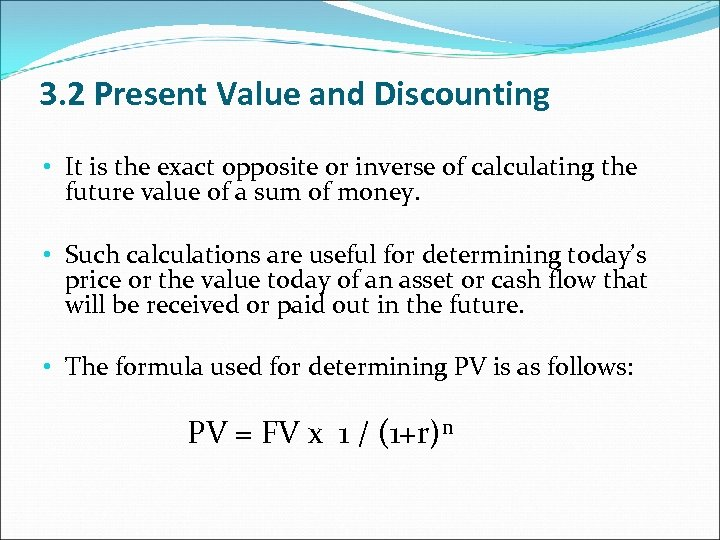3. 2 Present Value and Discounting • It is the exact opposite or inverse of calculating the future value of a sum of money. • Such calculations are useful for determining today’s price or the value today of an asset or cash flow that will be received or paid out in the future. • The formula used for determining PV is as follows: PV = FV x 1 / (1+r)n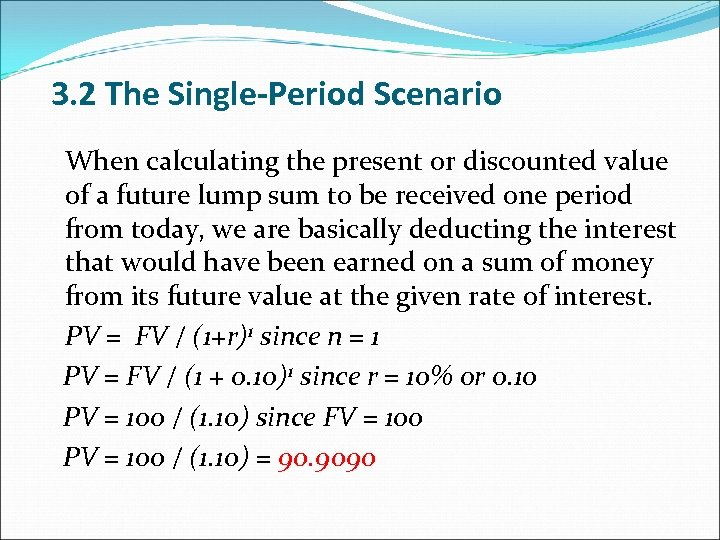3. 2 The Single-Period Scenario When calculating the present or discounted value of a future lump sum to be received one period from today, we are basically deducting the interest that would have been earned on a sum of money from its future value at the given rate of interest. PV = FV / (1+r)1 since n = 1 PV = FV / (1 + 0. 10)1 since r = 10% or 0. 10 PV = 100 / (1. 10) since FV = 100 PV = 100 / (1. 10) = 90. 9090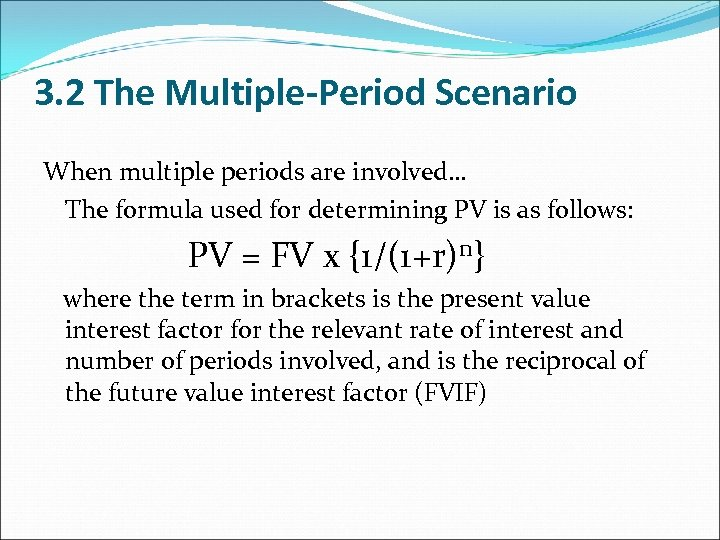3. 2 The Multiple-Period Scenario When multiple periods are involved… The formula used for determining PV is as follows: PV = FV x {1/(1+r)n} where the term in brackets is the present value interest factor for the relevant rate of interest and number of periods involved, and is the reciprocal of the future value interest factor (FVIF)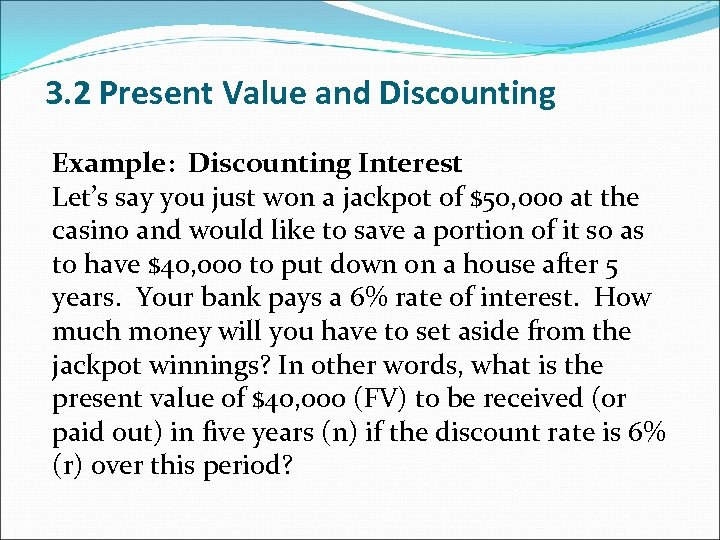3. 2 Present Value and Discounting Example: Discounting Interest Let’s say you just won a jackpot of \$50, 000 at the casino and would like to save a portion of it so as to have \$40, 000 to put down on a house after 5 years. Your bank pays a 6% rate of interest. How much money will you have to set aside from the jackpot winnings? In other words, what is the present value of \$40, 000 (FV) to be received (or paid out) in five years (n) if the discount rate is 6% (r) over this period?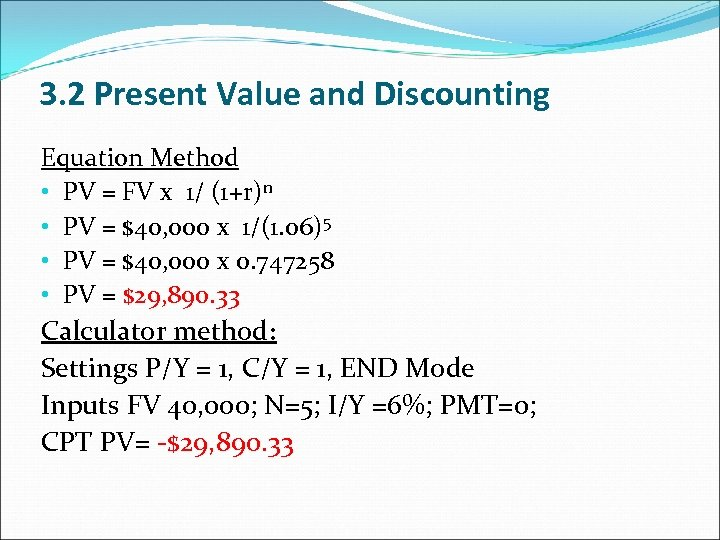3. 2 Present Value and Discounting Equation Method • PV = FV x 1/ (1+r)n • PV = \$40, 000 x 1/(1. 06)5 • PV = \$40, 000 x 0. 747258 • PV = \$29, 890. 33 Calculator method: Settings P/Y = 1, C/Y = 1, END Mode Inputs FV 40, 000; N=5; I/Y =6%; PMT=0; CPT PV= -\$29, 890. 33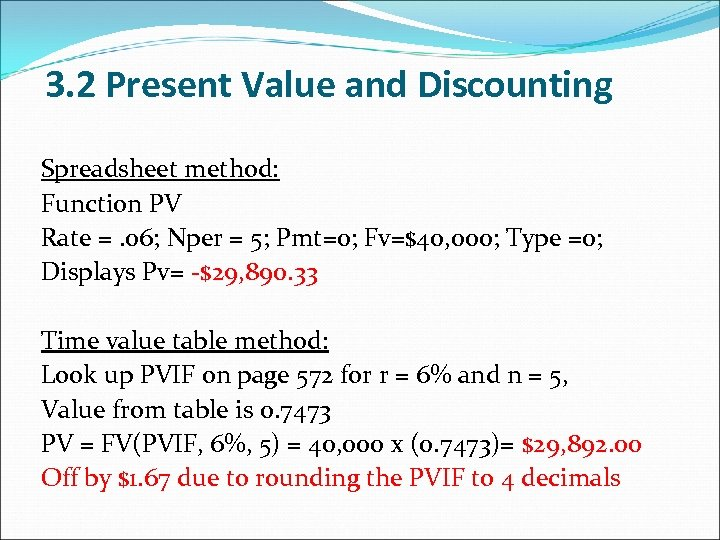3. 2 Present Value and Discounting Spreadsheet method: Function PV Rate =. 06; Nper = 5; Pmt=0; Fv=\$40, 000; Type =0; Displays Pv= -\$29, 890. 33 Time value table method: Look up PVIF on page 572 for r = 6% and n = 5, Value from table is 0. 7473 PV = FV(PVIF, 6%, 5) = 40, 000 x (0. 7473)= \$29, 892. 00 Off by \$1. 67 due to rounding the PVIF to 4 decimals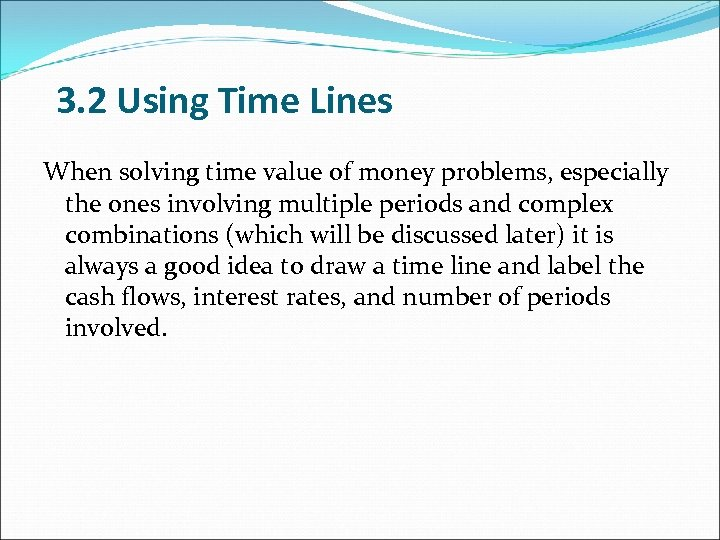3. 2 Using Time Lines When solving time value of money problems, especially the ones involving multiple periods and complex combinations (which will be discussed later) it is always a good idea to draw a time line and label the cash flows, interest rates, and number of periods involved.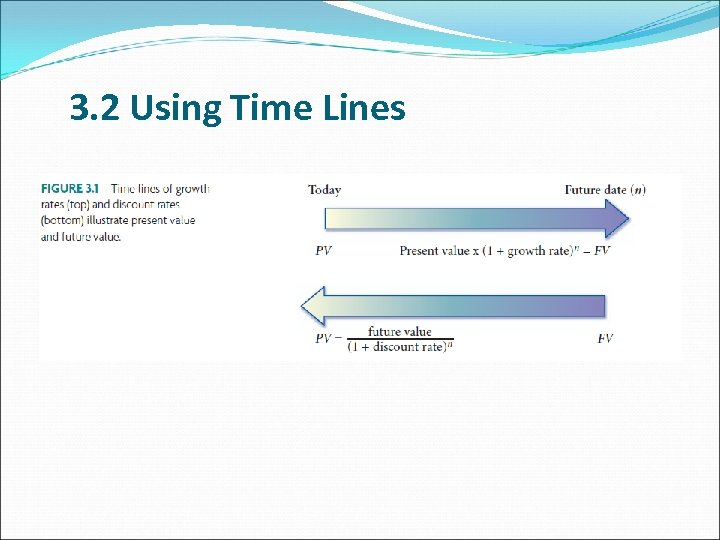3. 2 Using Time Lines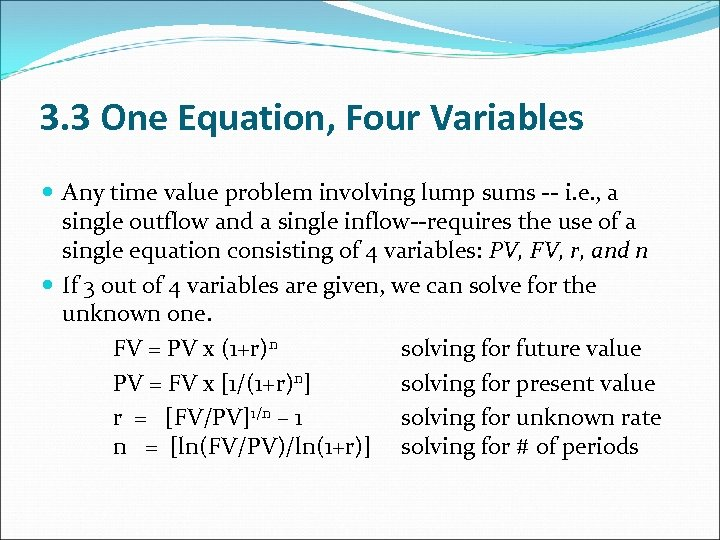3. 3 One Equation, Four Variables Any time value problem involving lump sums -- i. e. , a single outflow and a single inflow--requires the use of a single equation consisting of 4 variables: PV, FV, r, and n If 3 out of 4 variables are given, we can solve for the unknown one. FV = PV x (1+r)n solving for future value PV = FV x [1/(1+r)n] solving for present value r = [FV/PV]1/n – 1 solving for unknown rate n = [ln(FV/PV)/ln(1+r)] solving for # of periods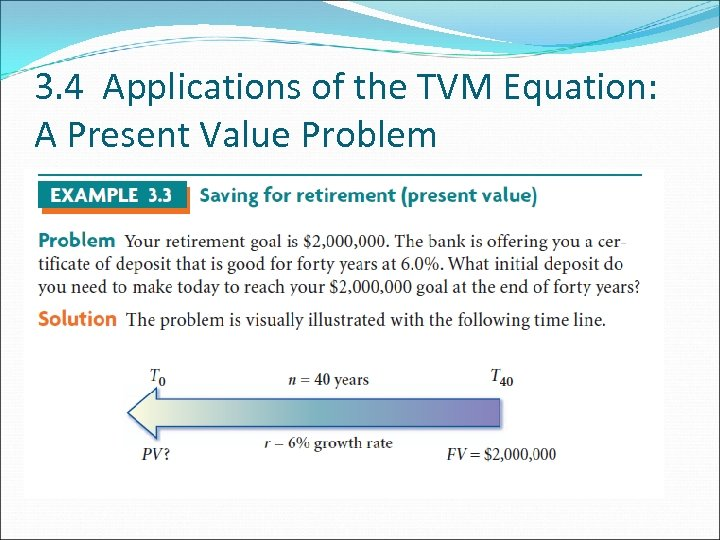3. 4 Applications of the TVM Equation: A Present Value Problem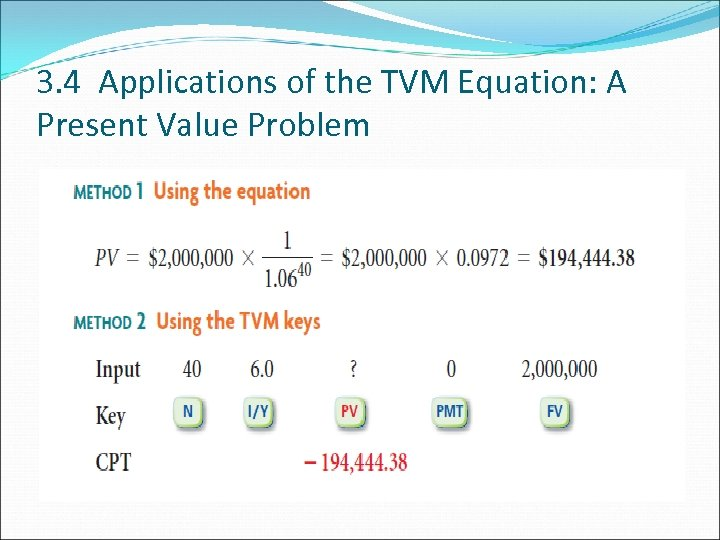3. 4 Applications of the TVM Equation: A Present Value Problem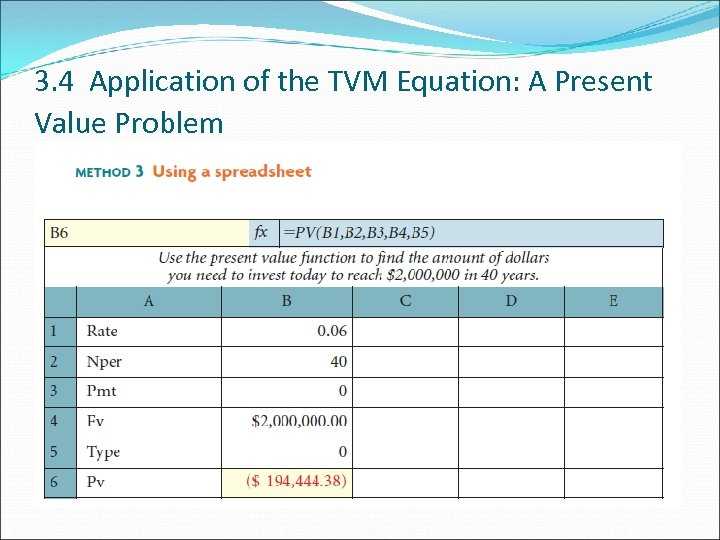3. 4 Application of the TVM Equation: A Present Value Problem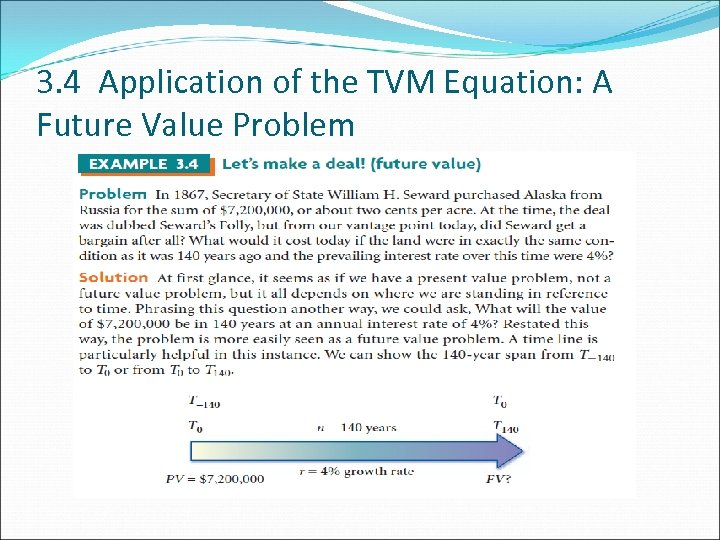3. 4 Application of the TVM Equation: A Future Value Problem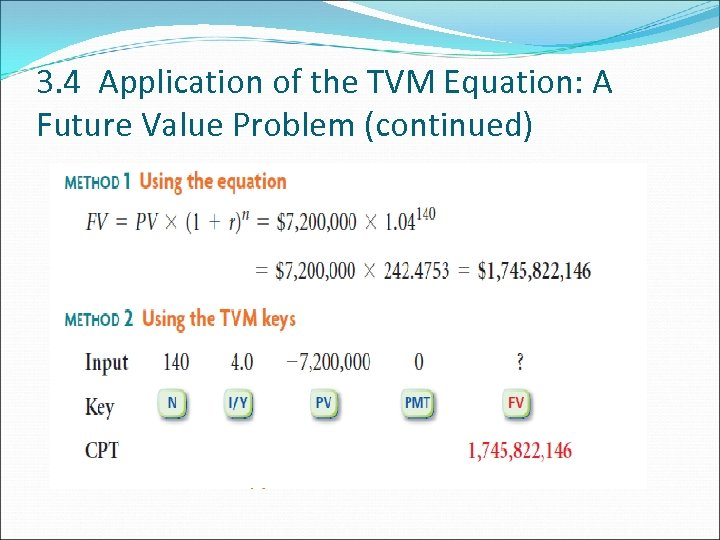3. 4 Application of the TVM Equation: A Future Value Problem (continued)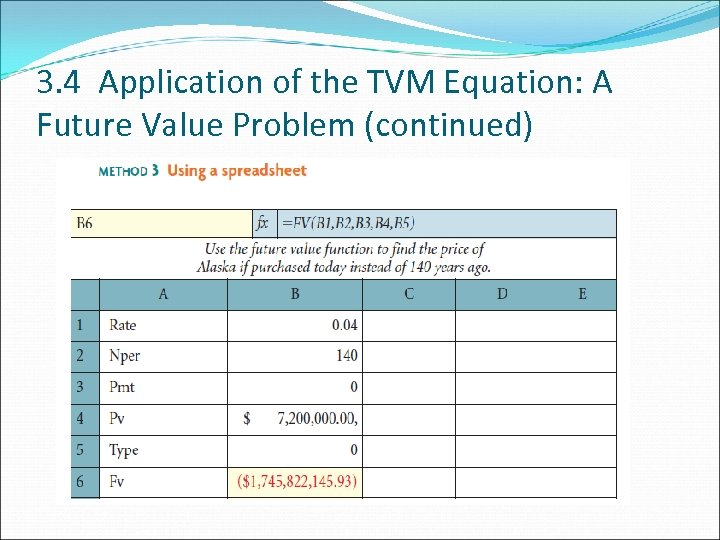3. 4 Application of the TVM Equation: A Future Value Problem (continued)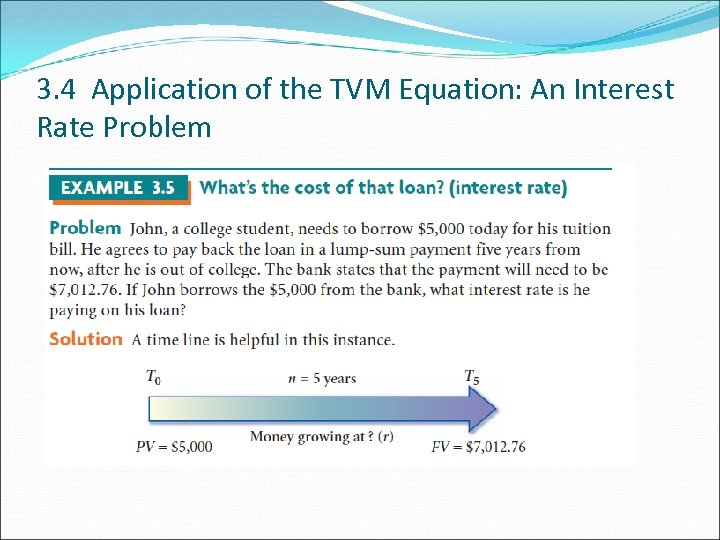3. 4 Application of the TVM Equation: An Interest Rate Problem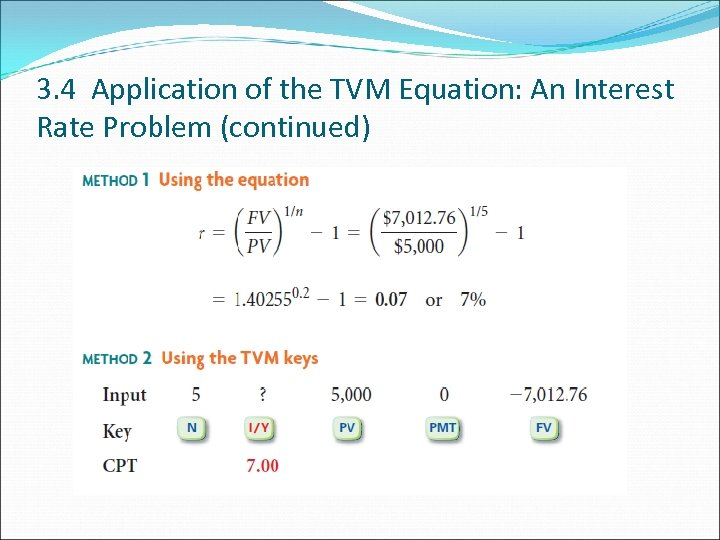3. 4 Application of the TVM Equation: An Interest Rate Problem (continued)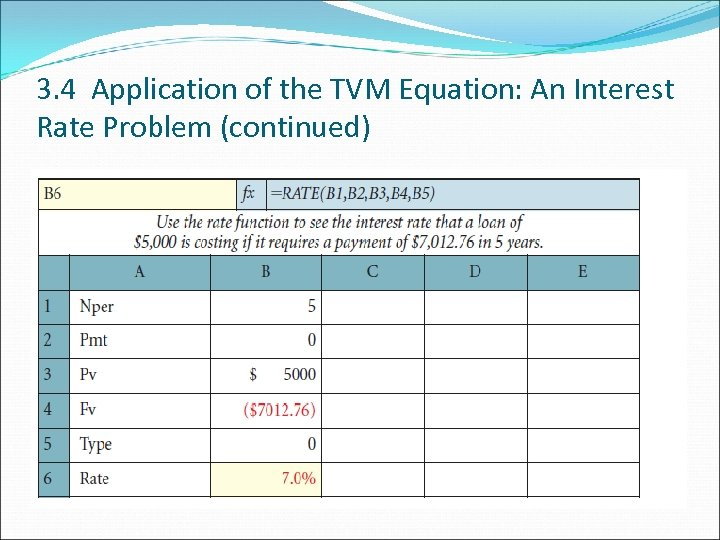3. 4 Application of the TVM Equation: An Interest Rate Problem (continued)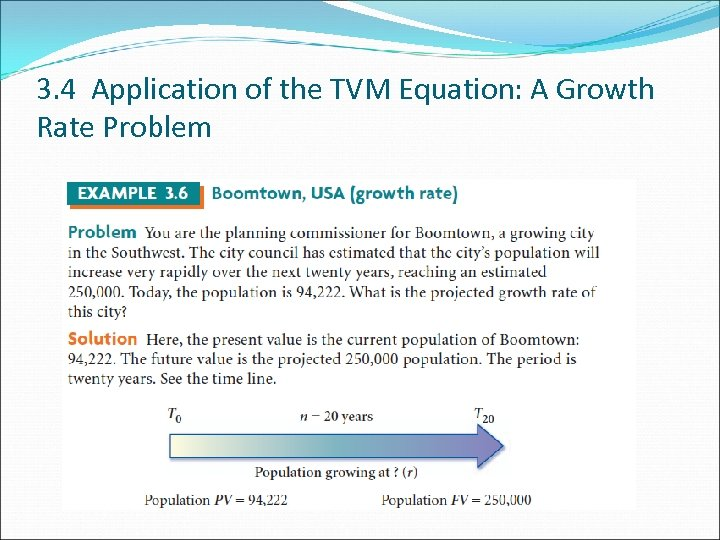3. 4 Application of the TVM Equation: A Growth Rate Problem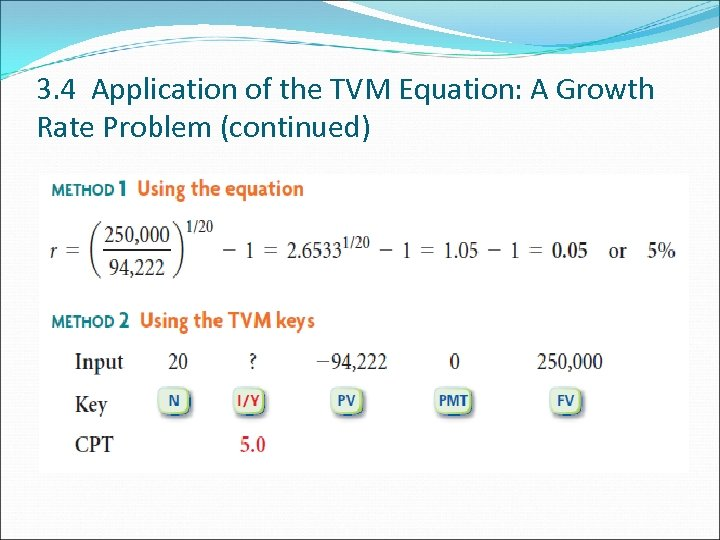3. 4 Application of the TVM Equation: A Growth Rate Problem (continued)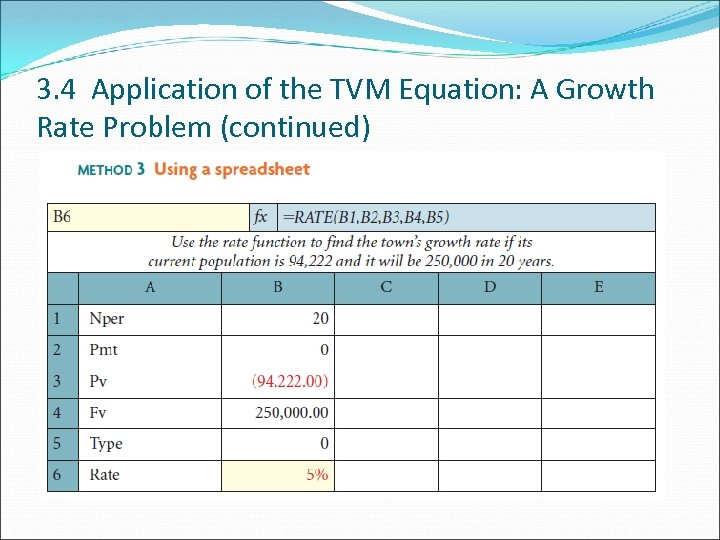3. 4 Application of the TVM Equation: A Growth Rate Problem (continued)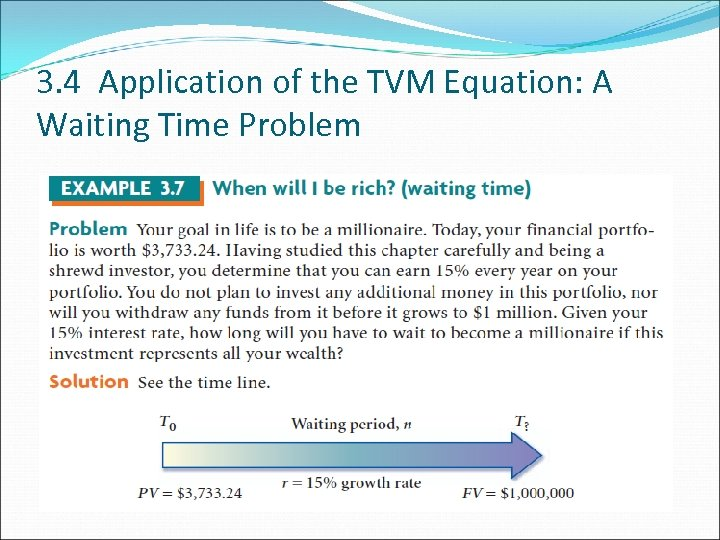3. 4 Application of the TVM Equation: A Waiting Time Problem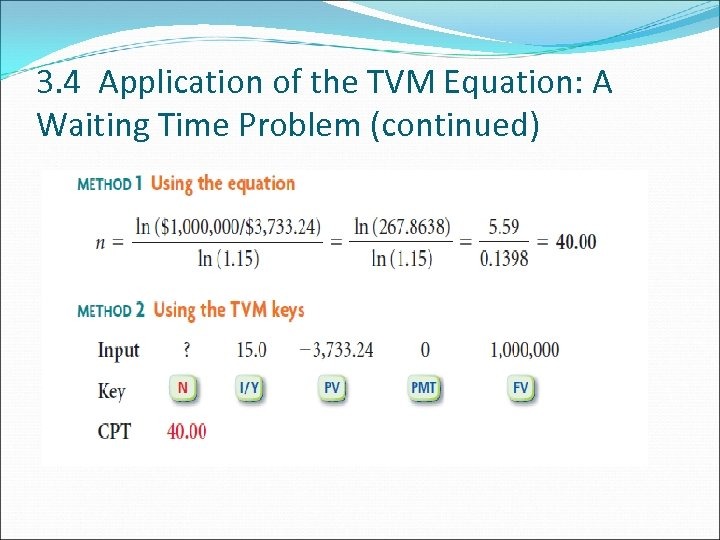3. 4 Application of the TVM Equation: A Waiting Time Problem (continued)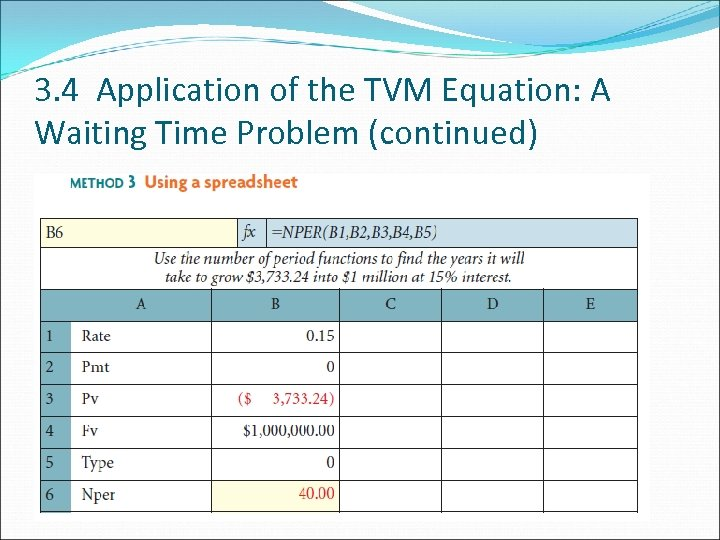3. 4 Application of the TVM Equation: A Waiting Time Problem (continued)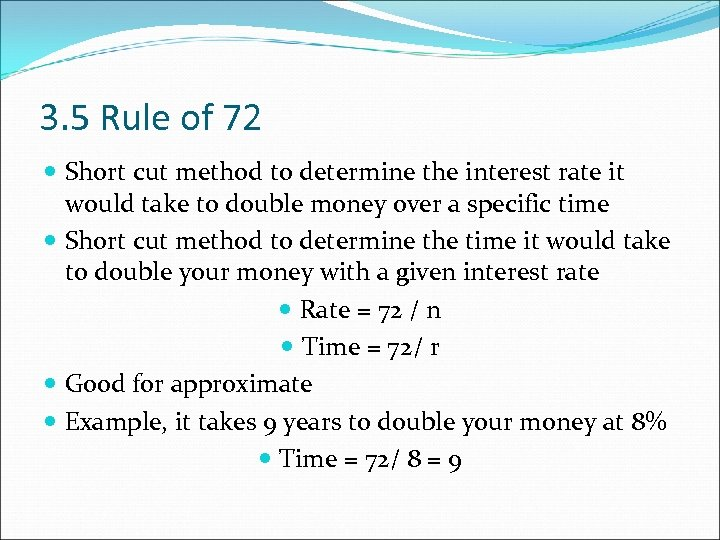3. 5 Rule of 72 Short cut method to determine the interest rate it would take to double money over a specific time Short cut method to determine the time it would take to double your money with a given interest rate Rate = 72 / n Time = 72/ r Good for approximate Example, it takes 9 years to double your money at 8% Time = 72/ 8 = 9